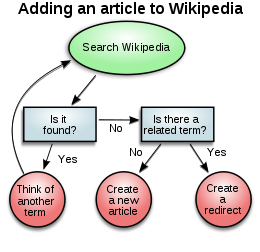# Diagram

﻿
DiagramSample flowchart representing the decision process to add a new article to Wikipedia.

A diagram is a two-dimensional geometric symbolic representation of information according to some visualization technique. Sometimes, the technique uses a three-dimensional visualization which is then projected onto the two-dimensional surface. The word graph is sometimes used as a synonym for diagram.

Today, most diagrams are generated using diagramming software.

## Overview

A diagram is a 2D geometric symbolic representation of information according to some visualization technique. Sometimes, the technique uses a 3D visualization which is then projected onto the 2D surface. The term diagram in common sense can have two meanings.

• visual information device : Like the term "illustration" the diagram is used as a collective term standing for the whole class of technical genres, including graphs, technical drawings and tables.
• specific kind of visual display : This is only the genre, that show qualitative data with shapes that are connected by lines, arrows, or other visual links.

In science the term is used in both ways. For example Anderson (1997) stated more generally: "diagrams are pictorial, yet abstract, representations of information, and maps, line graphs, bar charts, engineering blueprints, and architects' sketches are all examples of diagrams, whereas photographs and video are not". On the other hand Lowe (1993) defined diagrams as specifically "abstract graphic portrayals of the subject matter they represent".

In the specific sense diagrams and charts contrast computer graphics, technical illustrations, infographics, maps, and technical drawings, by show "abstract rather than literal representations of information". The essences of a diagram can be seen as:

• a form of visual formatting devices
• a display that do not show quantitative data, but rather relationships and abstract information
• with building blocks such as geometrical shapes connected by lines, arrows, or other visual links.

Or in Hall's (1996) words "diagrams are simplified figures, caricatures in a way, intended to convey essential meaning". These simplified figures are often based on set of rules. The basic shape according to White (1984) can be characterized in terms of "elegance, clarity, ease, pattern, simplicity, and validity". The elegance for a start is determined by whether or not the diagram is "the simplest and most fitting solution to a problem".

## Main diagram types

There are at least the following types of diagrams:

• Graph-based diagrams: these take a collection of items and relationships between them, and express them by giving each item a 2D position, while the relationships are expressed as connections between the items or overlaps between the items; examples of such techniques:
• Chart-like diagram techniques, which display a relationship between two variables that take either discrete or a continuous ranges of values; examples:
• Other types of diagrams, e.g.,

Thousands of diagram techniques exist. Some more examples follow.

## Specific diagram types

### A–D

A
B
C
D

List of modeling languages

### E–H

E
• Entity-Relationship diagram (ERD)
• Event-driven process chain
• Euler diagram
• Eye diagram - a diagram of a received telecommunications signal
• Express-G
• Extended Functional Flow Block Diagram (EFFBD)
F
G
H

### I–L

I
J
K
L
• Line of balance

M
N
O
P

### R–U

R
• Requirement Diagram Used in SysML
• Rich Picture
S
T
U

### V–Z

V
W
• Warnier-Orr
Y

Wikimedia Foundation. 2010.

Synonyms:

### Look at other dictionaries:

• diagram — di‧a‧gram [ˈdaɪəgræm] noun [countable] a drawing or plan that shows where something is, what it looks like, or how it works: • See the attached diagram for details on current market projections. • a diagram of the electrical system ˈblock… …   Financial and business terms

• diagram — [dī′ə gram΄] n. [Gr diagramma < diagraphein, to mark out by lines, draw < dia (see DIA ) + graphein, to write (see GRAPHIC)] 1. a geometric figure, used to illustrate a mathematical statement, proof, etc. 2. a sketch, drawing, or plan that… …   English World dictionary

• Diagram — Di a*gram, n. [Gr. ?, fr. ? to mark out by lines; dia through + ? to draw, write: cf. F. diagramme. See {Graphic}.] 1. (Geom.) A figure or drawing made to illustrate a statement, or facilitate a demonstration; a plan. [1913 Webster] 2. Any simple …   The Collaborative International Dictionary of English

• Diagram — Di a*gram, v. t. To put into the form of a diagram. [1913 Webster] …   The Collaborative International Dictionary of English

• diagram — n outline, draft, tracing, sketch, delineation, plot, blueprint (see under SKETCH vb) Analogous words: design, *plan, plot, scheme diagram vb outline, plot, blueprint, draft, trace, *sketch, delineate Analogous words: design, plan, plot, scheme… …   New Dictionary of Synonyms

• diagram — verb, meaning ‘to represent by means of a diagram’ (the outline is diagrammed on the cover), has the forms diagrammed, diagramming, diagrammatic. In AmE the verbal forms are often diagramed, diagraming …   Modern English usage

• diagram — ► NOUN ▪ a drawing giving a schematic representation of the appearance or structure of something. ► VERB (diagrammed, diagramming; US diagramed, diagraming) ▪ represent by means of a diagram. DERIVATIVES diagrammatic adjective …   English terms dictionary

• diagram — index blueprint, characterize, contour (outline), delineate, delineation, depict, design (construction plan) …   Law dictionary

• diagram — (n.) 1610s, from Fr. diagramme, from L. diagramma, from Gk. diagramma geometric figure, that which is marked out by lines, from diagraphein mark out by lines, delineate, from dia across, out (see DIA (Cf. dia )) + graphein write, mark, draw (see… …   Etymology dictionary

• diagram — An official plan either for labeling letter cases and sorting racks or for loading mail onto a vehicle, airplane, or rail container …   Glossary of postal terms

• diagram — [n] drawing, sketch of form or plan big picture*, blueprint, chart, description, design, draft, figure, floor plan, game, game plan, ground plan, layout, outline, perspective, representation, rough draft; concepts 625,660 …   New thesaurus